### Example 53.1 Engine Exhaust Emissions

Investigators studied the exhaust emissions of a one-cylinder engine (Brinkman, 1981). The SAS data set Gas contains the results data. The dependent variable, NOx, measures the concentration, in micrograms per joule, of nitric oxide and nitrogen dioxide normalized by the amount of work of the engine. The independent variable, E, is a measure of the richness of the air and fuel mixture.

data Gas;
input NOx E @@;
format NOx f3.1;
format E f3.1;
datalines;
4.818  0.831   2.849  1.045
3.275  1.021   4.691  0.97
4.255  0.825   5.064  0.891
2.118  0.71    4.602  0.801
2.286  1.074   0.97   1.148
3.965  1       5.344  0.928
3.834  0.767   1.99   0.701
5.199  0.807   5.283  0.902
3.752  0.997   0.537  1.224
1.64   1.089   5.055  0.973
4.937  0.98    1.561  0.665
;


The following PROC SGPLOT statements produce the simple scatter plot of these data displayed in Output 53.1.1.


proc sgplot data=Gas;
scatter x=E y=NOx;
run;


Output 53.1.1: Scatter Plot of the Gas Data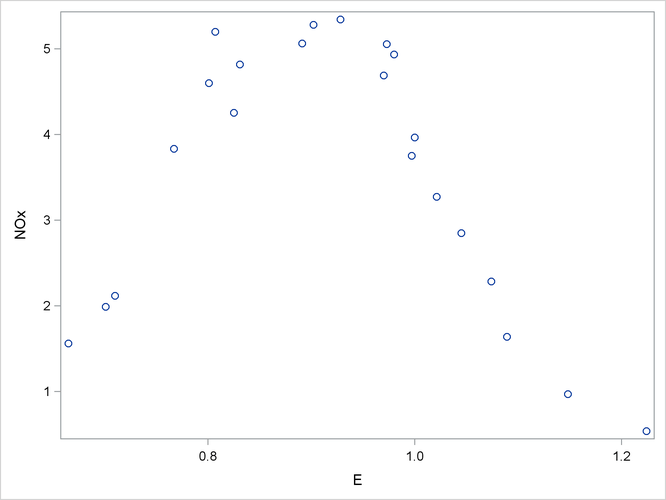The following statements fit two loess models for these data. Because this is a small data set, it is reasonable to do direct fitting at every data point. As there is substantial curvature in the data, quadratic local polynomials are used. An ODS OUTPUT statement creates two output data sets containing the Output Statistics and Fit Summary tables.

ods graphics on;

proc loess data=Gas;
ods output OutputStatistics = GasFit
FitSummary=Summary;
model NOx = E / degree=2 select=AICC(steps) smooth = 0.6 1.0
direct alpha=.01 all details;
run;

ods graphics off;


Output 53.1.2: Fit Summary Table

The LOESS Procedure
Selected Smoothing Parameter: 0.6
Dependent Variable: NOx

Fit Summary
Fit Method Direct
Number of Observations 22
Degree of Local Polynomials 2
Smoothing Parameter 0.60000
Points in Local Neighborhood 13
Residual Sum of Squares 1.71852
Trace[L] 6.42184
GCV 0.00708
AICC -0.45637
AICC1 -9.39715
Delta1 15.12582
Delta2 14.73089
Equivalent Number of Parameters 5.96950
Lookup Degrees of Freedom 15.53133
Residual Standard Error 0.33707

The Fit Summary table for smoothing parameter value 0.6, shown in Output 53.1.2, records the fitting parameters specified and some overall fit statistics. See the section Smoothing Matrix for a definition of the smoothing matrix, and the sections Model Degrees of Freedom and Statistical Inference and Lookup Degrees of Freedom for definitions of the statistics that appear this table.

The Output Statistics table for smoothing parameter value 0.6 is shown in Output 53.1.3. Note that, because the ALL option is specified in the MODEL statement, this table includes all the relevant optional columns. Furthermore, because the ALPHA=0.01 option is specified in the MODEL statement, the confidence limits in this table are 99% limits.

Output 53.1.3: Output Statistics Table

The LOESS Procedure
Selected Smoothing Parameter: 0.6
Dependent Variable: NOx

Output Statistics
Obs E NOx Predicted NOx Estimated
Prediction
Std Deviation
Residual t Value 99% Confidence Limits
1 0.8 4.8 4.87377 0.15528 -0.05577 -0.36 4.41841 5.32912
2 1.0 2.8 2.81984 0.15380 0.02916 0.19 2.36883 3.27085
3 1.0 3.3 3.48153 0.15187 -0.20653 -1.36 3.03617 3.92689
4 1.0 4.7 4.73249 0.13923 -0.04149 -0.30 4.32419 5.14079
5 0.8 4.3 4.82305 0.15278 -0.56805 -3.72 4.37503 5.27107
6 0.9 5.1 5.18561 0.19337 -0.12161 -0.63 4.61855 5.75266
7 0.7 2.1 2.51120 0.15528 -0.39320 -2.53 2.05585 2.96655
8 0.8 4.6 4.48267 0.15285 0.11933 0.78 4.03444 4.93089
9 1.1 2.3 2.12619 0.16683 0.15981 0.96 1.63697 2.61541
10 1.1 1.0 0.97120 0.18134 -0.00120 -0.01 0.43942 1.50298
11 1.0 4.0 4.09987 0.13477 -0.13487 -1.00 3.70467 4.49507
12 0.9 5.3 5.31258 0.17283 0.03142 0.18 4.80576 5.81940
13 0.8 3.8 3.84572 0.14929 -0.01172 -0.08 3.40794 4.28350
14 0.7 2.0 2.26578 0.16712 -0.27578 -1.65 1.77571 2.75584
15 0.8 5.2 4.58394 0.15363 0.61506 4.00 4.13342 5.03445
16 0.9 5.3 5.24741 0.19319 0.03559 0.18 4.68089 5.81393
17 1.0 3.8 4.16979 0.13478 -0.41779 -3.10 3.77457 4.56502
18 1.2 0.5 0.53059 0.32170 0.00641 0.02 -0.41278 1.47397
19 1.1 1.6 1.83157 0.17127 -0.19157 -1.12 1.32933 2.33380
20 1.0 5.1 4.66733 0.13735 0.38767 2.82 4.26456 5.07010
21 1.0 4.9 4.52385 0.13556 0.41315 3.05 4.12632 4.92139
22 0.7 1.6 1.19888 0.26774 0.36212 1.35 0.41375 1.98401

Output 53.1.4: Output Statistics Table

Optimal Smoothing Criterion
AICC Smoothing
Parameter
-0.45637 0.60000

The combination of the options SELECT=AICC and SMOOTH=0.6 1 in the MODEL statement specifies that PROC LOESS fit models with smoothing parameters of 0.6 and 1 and select the model that yields the smaller value of the AICC statistic. The Smoothing Criterion shown in Output 53.1.4 shows that PROC LOESS selects the model with smoothing parameter value 0.6 as it yields the smaller value of the AICC statistic.

With ODS Graphics enabled, PROC LOESS produces a panel of fit plots whenever you specify the SMOOTH= option in the MODEL statement. These fit plots include confidence limits if you additionally specify the CLM option in the MODEL statement.

Output 53.1.5: Loess Fits with 99% Confidence Limits for the Gas Data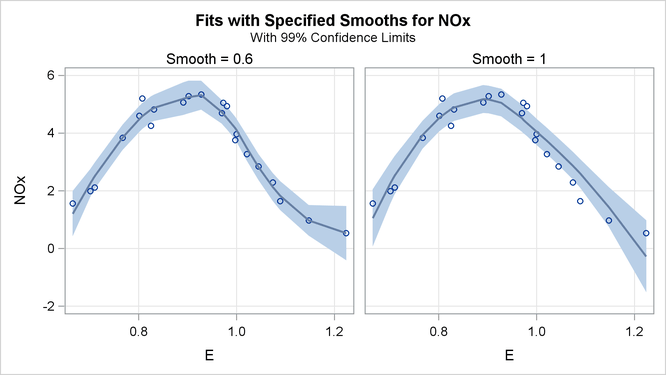Output 53.1.5 shows the Fit Panel that displays the fitted models with 99% confidence limits overlaid on scatter plots of the data.

Based on the AICC criterion, the model with smoothing parameter 0.6 is preferred. You can address the question of whether the differences between these models are significant using analysis of variance. You do this by using the model with smoothing parameter value 1 as the null model.

The statistic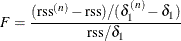has a distribution that is well approximated by an F distribution with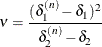numerator degrees of freedom anddenominator degrees of freedom (Cleveland and Grosse, 1991). Here quantities with superscript n refer to the null model, rss is the residual sum of squares, and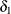,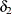, andare defined in the section Statistical Inference and Lookup Degrees of Freedom.

The Fit Summary tables contain the information needed to carry out such an analysis. These tables have been captured in the output data set named Summary by using an ODS OUTPUT statement. The following statements extract the relevant information from this data set and carry out the analysis of variance:

data h0 h1;
set Summary(keep=SmoothingParameter Label1 nValue1
where=(Label1 in ('Residual Sum of Squares','Delta1',
'Delta2','Lookup Degrees of Freedom')));
if SmoothingParameter = 1 then output h0;
else output h1;
run;

proc transpose data=h0(drop=SmoothingParameter Label1) out=h0;
run;

data h0(drop=_NAME_);
set h0;
Col2 = delta1Null
Col3 = delta2Null;
run;

proc transpose data=h1(drop=SmoothingParameter Label1) out=h1;
run;

data h1(drop=_NAME_);
set h1;
rename Col1 = RSS     Col2 = delta1
Col3 = delta2  Col4 = rho;
run;

data ftest;
merge h0 h1;
nu = (delta1Null - delta1)**2 / (delta2Null - delta2);
FValue = Numerator / Denominator;
PValue = 1 - ProbF(FValue, nu, rho);
label nu     = 'Num DF'   rho    = 'Den DF'
FValue = 'F Value'  PValue = 'Pr > F';
run;

proc print data=ftest label;
var nu rho Numerator Denominator FValue PValue;
format nu rho FValue 7.2 PValue 6.4;
run;


The results are shown in Output 53.1.6.

Output 53.1.6: Test ANOVA for Loess Models of Gas Data

Obs Num DF Den DF Numerator Denominator F Value Pr > F
1 2.67 15.53 1.05946 0.11362 9.32 0.0012

The small p-value confirms that the fit with smoothing parameter value 0.6 is significantly different from the loess model with smoothing parameter value 1.

Alternatively, you can use the OUTPUT statement to generate the statistics you want to include in the output data set. The following statements produce essentially the same results as the ODS OUTPUT statement does, except all the statistics for each of the two smoothing parameter values are included because the SELECT= option is not specified in the MODEL statement. In addition, with the ROW option specified, the output data set is arranged in rowwise format which enables you to compare statistics side-by-side for a sequence of smoothing values. The ALL option after the slash produces all the statistics (predicted values, residual values, standard errors of the mean predicted values, t statistics, and the lower and upper parts of% confidence limits on the mean predicted value). All these requested statistics are given their respective default names in the output data set except the predicted value. The P=PREDVAL option causes the name for the predicted value to start with predval.

proc loess data=Gas;
model NOx = E / degree=2 smooth = 0.6 1.0
direct alpha=.01;
output out=GasFit p=predval /all row;
run;